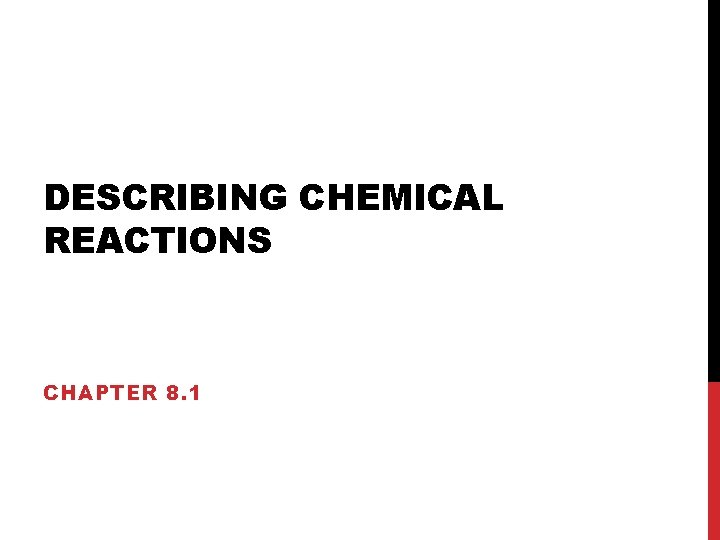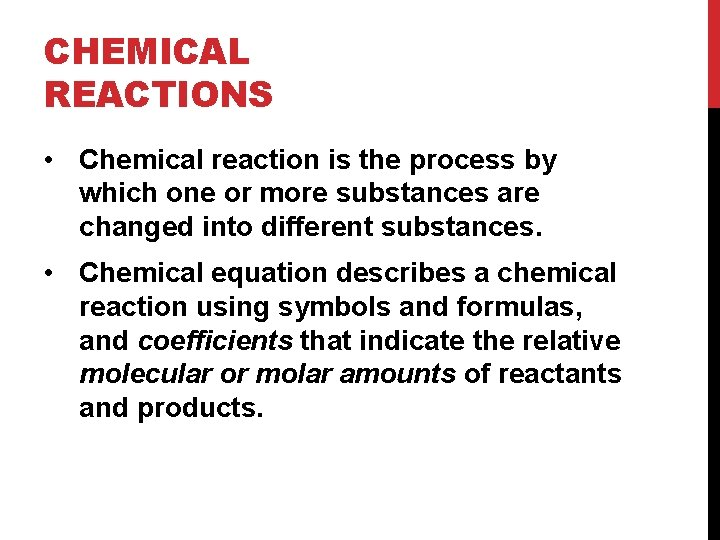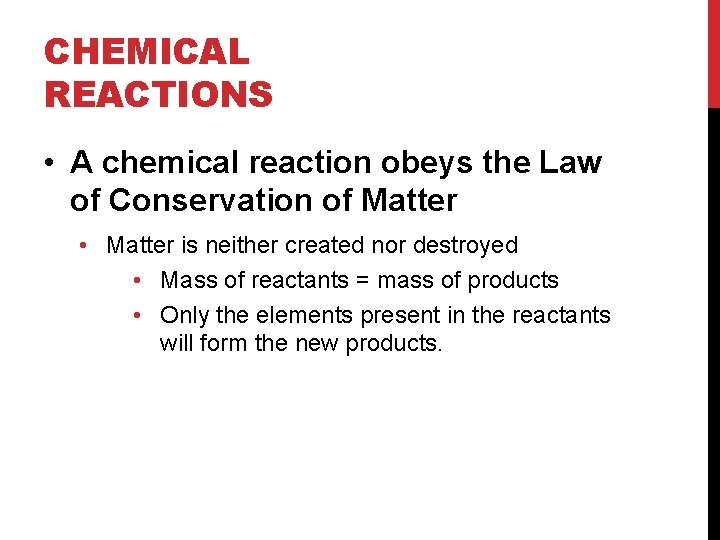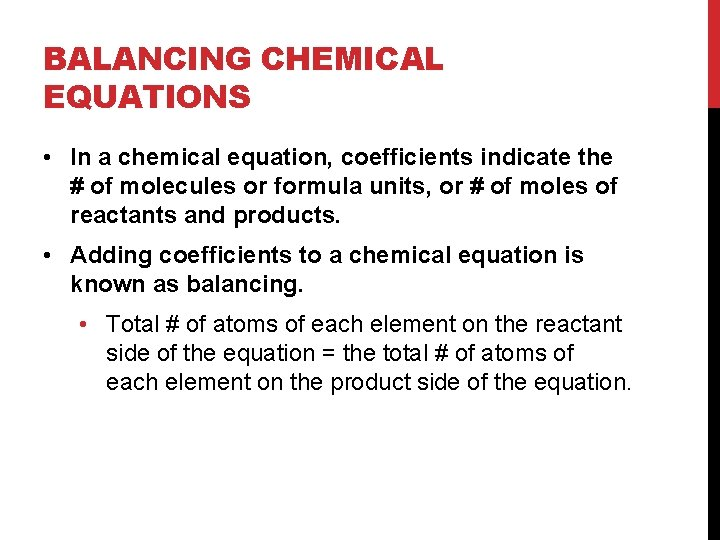# DESCRIBING CHEMICAL REACTIONS CHAPTER 8 1 CHEMICAL REACTIONS

• Slides: 6DESCRIBING CHEMICAL REACTIONS CHAPTER 8. 1CHEMICAL REACTIONS • Chemical reaction is the process by which one or more substances are changed into different substances. • Chemical equation describes a chemical reaction using symbols and formulas, and coefficients that indicate the relative molecular or molar amounts of reactants and products.CHEMICAL REACTIONS • A chemical reaction obeys the Law of Conservation of Matter • Matter is neither created nor destroyed • Mass of reactants = mass of products • Only the elements present in the reactants will form the new products.INDICATIONS OF A CHEMICAL REACTION • Evolution of energy in the form of heat and/or light • Color change • Production of a gas (bubbles). • Formation of a precipitate • A precipitate is a solid that forms when 2 solutions are mixed.BALANCING CHEMICAL EQUATIONS • In a chemical equation, coefficients indicate the # of molecules or formula units, or # of moles of reactants and products. • Adding coefficients to a chemical equation is known as balancing. • Total # of atoms of each element on the reactant side of the equation = the total # of atoms of each element on the product side of the equation.HOW TO BALANCE A CHEMICAL EQUATION 1. Count atoms of all elements that appear on the reactant and product side of the equation—make an inventory list. 2. Add coefficients in front of those chemical formulas whose elements need to be balanced. 1. Never change subscripts!!!! 2. Always balance elements other than oxygen and hydrogen first. 3. Leave hydrogen and oxygen for last. 3. Check your work—count all atoms again to ensure equation is balanced. 4. You want the lowest ratio of coefficients!